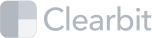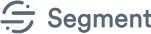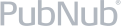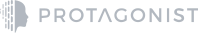# Understanding TF-ID: A Simple Introduction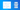TF-IDF (term frequency-inverse document frequency) is a statistical measure that evaluates how relevant a word is to a document in a collection of documents.

This is done by multiplying two metrics: how many times a word appears in a document, and the inverse document frequency of the word across a set of documents.

It has many uses, most importantly in automated text analysis, and is very useful for scoring words in machine learning algorithms for Natural Language Processing (NLP).

TF-IDF was invented for document search and information retrieval. It works by increasing proportionally to the number of times a word appears in a document, but is offset by the number of documents that contain the word. So, words that are common in every document, such as this, what, and if, rank low even though they may appear many times, since they don’t mean much to that document in particular.

However, if the word Bug appears many times in a document, while not appearing many times in others, it probably means that it’s very relevant. For example, if what we’re doing is trying to find out which topics some NPS responses belong to, the word Bug would probably end up being tied to the topic Reliability, since most responses containing that word would be about that topic.

## How is TF-IDF calculated?

TF-IDF for a word in a document is calculated by multiplying two different metrics:

• The term frequency of a word in a document. There are several ways of calculating this frequency, with the simplest being a raw count of instances a word appears in a document. Then, there are ways to adjust the frequency, by length of a document, or by the raw frequency of the most frequent word in a document.
• The inverse document frequency of the word across a set of documents. This means, how common or rare a word is in the entire document set. The closer it is to 0, the more common a word is. This metric can be calculated by taking the total number of documents, dividing it by the number of documents that contain a word, and calculating the logarithm.
• So, if the word is very common and appears in many documents, this number will approach 0. Otherwise, it will approach 1.

Multiplying these two numbers results in the TF-IDF score of a word in a document. The higher the score, the more relevant that word is in that particular document.

To put it in more formal mathematical terms, the TF-IDF score for the word t in the document d from the document set D is calculated as follows:Where:## Why is TF-IDF used in Machine Learning?

Machine learning with natural language is faced with one major hurdle – its algorithms usually deal with numbers, and natural language is, well, text. So we need to transform that text into numbers, otherwise known as text vectorization. It’s a fundamental step in the process of machine learning for analyzing data, and different vectorization algorithms will drastically affect end results, so you need to choose one that will deliver the results you’re hoping for.

Once you’ve transformed words into numbers, in a way that’s machine learning algorithms can understand, the TF-IDF score can be fed to algorithms such as Naive Bayes and Support Vector Machines, greatly improving the results of more basic methods like word counts.

Why does this work? Simply put, a word vector represents a document as a list of numbers, with one for each possible word of the corpus. Vectorizing a document is taking the text and creating one of these vectors, and the numbers of the vectors somehow represent the content of the text. TF-IDF enables us to gives us a way to associate each word in a document with a number that represents how relevant each word is in that document. Then, documents with similar, relevant words will have similar vectors, which is what we are looking for in a machine learning algorithm.

## Applications of TF-IDF

Determining how relevant a word is to a document, or TD-IDF, is useful in many ways, for example:

• Information retrieval

TF-IDF was invented for document search and can be used to deliver results that are most relevant to what you’re searching for. Imagine you have a search engine and somebody looks for LeBron. The results will be displayed in order of relevance. That’s to say the most relevant sports articles will be ranked higher because TF-IDF gives the word LeBron a higher score.

It’s likely that every search engine you have ever encountered uses TF-IDF scores in its algorithm.

• Keyword Extraction

TF-IDF is also useful for extracting keywords from text. How? The highest scoring words of a document are the most relevant to that document, and therefore they can be considered keywords for that document. Pretty straightforward.

## Final words

It’s useful to understand how TF-IDF works so that you can gain a better understanding of how machine learning algorithms function. While machine learning algorithms traditionally work better with numbers, TF-IDF algorithms help them decipher words by allocating them a numerical value or vector. This has been revolutionary for machine learning, especially in fields related to NLP such as text analysis.

In text analysis with machine learning, TF-IDF algorithms help sort data into categories, as well as extract keywords. This means that simple, monotonous tasks, like tagging support tickets or rows of feedback and inputting data can be done in seconds.

Every wondered how Google can serve up information related to your search in mere seconds? Well, now you know. Text vectorization transforms text within documents into numbers, so TF-IDF algorithms can rank articles in order of relevance.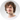May 10th, 2019

## Posts you might like...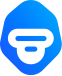## Text Analysis with Machine Learning

Turn tweets, emails, documents, webpages and more into actionable data. Automate business processes and save hours of manual data processing.